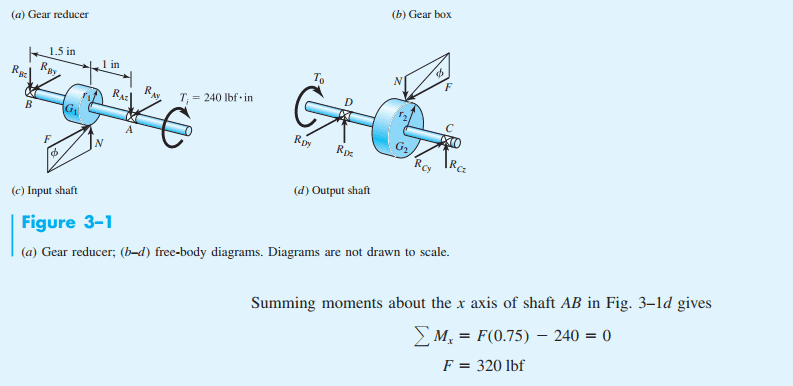# Find the reaction forces of shaft AB

• Engineering
• LT72884
When you open your hand, the moment goes away and the object stays in the same place (unless you keep applying a moment). Similarly, when you have two moments, there is a resultant moment that combines the two individual moments.f

#### LT72884

Homework Statement
find reaction forces of shaft AB
Relevant Equations
NAGiven: r1 = 0.75 r2 = 1.5
Ok, i have done statics and dynamics, but this book does things differently than the way i accomplished it in the previous classes. This is for mechanical engineering design class.

Here is my question. They take sum of the moments along X, which goes through the center of the rod, but i can't seem to see where they are taking the moment AT? Also where does (0.75) came from? I know its the radius of the gear on the shaft, but why are they using it for F? That is not even in the x direction. i do not see why and where they took the moment at.

thanks:)

Last edited by a moderator:
Determine the reactions of simply supported, overhanging and cantilever beams; Calculate and draw the shearing force and bending moment diagrams of beams ... would be subjected to a torque, it would be called and treated as a shaft.
this doesn't seem to help me figure out where they are taking the moment at in the picture. They say the sum of the moments about x, but i don't see x and i don't see why they are bringing in the gear dimensions to anser the question

this doesn't seem to help me figure out where they are taking the moment at in the picture. They say the sum of the moments about x, but i don't see x and i don't see why they are bringing in the gear dimensions to anser the question
Since they show the directions of axes y and z, we can assume that each axis x is colineal with the rotational axis of each of the shafts.

The book is trying to calculate the force on the contacting teeth of the gears from the value of the input moment trying to twist the input shaft, which acts about its axis.
That equation shows the balance (Mx=0) between reactive torque of F times r1 and input torque.

The problem is that something is not correct in the book, because shaft AB (linked to gear of r1=0.75 inch) is represented in figure c rather than d.

The torque exerted by each gear on its shaft is proportional to its radius (r1 for input shaft and r2 for output shaft).
The output torque should be greater than the input torque in the same proportion in which r1 and r2 are different (as F is the same for both).

Last edited:
im glad I am not the only one who noticed the issue with the book with the typo.

I thought when we took a moment, it was force(distance) why is it not force time the distance from the gear to the end of the shaft? why is it force time the distance of the gear, IE r1?

thanks

... I thought when we took a moment, it was force(distance) why is it not force time the distance from the gear to the end of the shaft?
Please, note that you have force and distance(s) acting on the three planes.
Force F is trying to bend the shaft and induces both reaction forces at the bearings.
Same for force N.
As force F is the only acting off the axis of rotation of the shaft, it also induces a torsional moment.

Using force times distance, you can calculate any of the two bending moments and the torsional moment.
Each of those moments acts about a different axis of rotation.

•LT72884
The shafts are spinning at constant speeds so the sum of moments about their longitudinal axes must be =0 (no acceleration). This is stated in the problem. Summing the moments along this axis means accounting for all forces and moments that would act in such a direction as to cause the shaft to rotate (or prevent it from rotating - if counteracting another moment). Looking at shaft AB, there are two moments acting on it (along x-axis): (240in-lb) and (-F*r1) with r1=0.75 given. These must sum to 0.

You might need to familiarize yourself with how moments are specified. For example, with your fist closed and thumb up, a moment in the direction of your thumb corresponds to a rotation following the curl of your fingers.